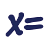Games
Problems
Go Pro!

Reference > Mathematics > AlgebraThe quadratic formula begins with the concept of completing a square.Completing a square as a step in understanding the quadratic formulaHow to use completing the square as a means for solving a quadratic equationWhat happens when the coefficient of x squared isn't a perfect square?What happens if the number you add to complete the square is itself not a perfect square?Finally, we put everything we've done together in one great big formula!Like us on Facebook to get updates about new resources